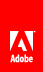# Assembling and transforming XML objects

Flash Player 9 and later, Adobe AIR 1.0 and later

 Use the prependChild() method or the appendChild() method to add a property to the beginning or end of an XML object’s list of properties, as the following example shows: ```var x1:XML =

Line 1

var x2:XML =

Line 2

var x:XML = x = x.appendChild(x1); x = x.appendChild(x2); x = x.prependChild(

Line 0

); // x ==

Line 0

Line 1

Line 2

``` Use the insertChildBefore() method or the insertChildAfter() method to add a property before or after a specified property, as follows: ```var x:XML =

Paragraph 1

Paragraph 2

var newNode:XML =

Paragraph 1.5

x = x.insertChildAfter(x.p, newNode) x = x.insertChildBefore(x.p,

Paragraph 1.75

)``` As the following example shows, you can also use curly brace operators ( { and } ) to pass data by reference (from other variables) when constructing XML objects: ```var ids:Array = [121, 122, 123]; var names:Array = [["Murphy","Pat"], ["Thibaut","Jean"], ["Smith","Vijay"]] var x:XML = new XML(""); for (var i:int = 0; i < 3; i++) { var newnode:XML = new XML(); newnode = {names[i]} {names[i]} ; x = x.appendChild(newnode) }``` You can assign properties and attributes to an XML object by using the = operator, as in the following: ```var x:XML = Smith x.firstname = "Jean"; x.@id = "239";``` This sets the XML object x to the following: ``` Smith Jean ``` You can use the + and += operators to concatenate XMLList objects: ```var x1:XML = test1 var x2:XML = test2 var xList:XMLList = x1 + x2; xList += test3``` This sets the XMLList object xList to the following: ```test1 test2 test3```Twitter™ and Facebook posts are not covered under the terms of Creative Commons.// Ethnio survey code removed